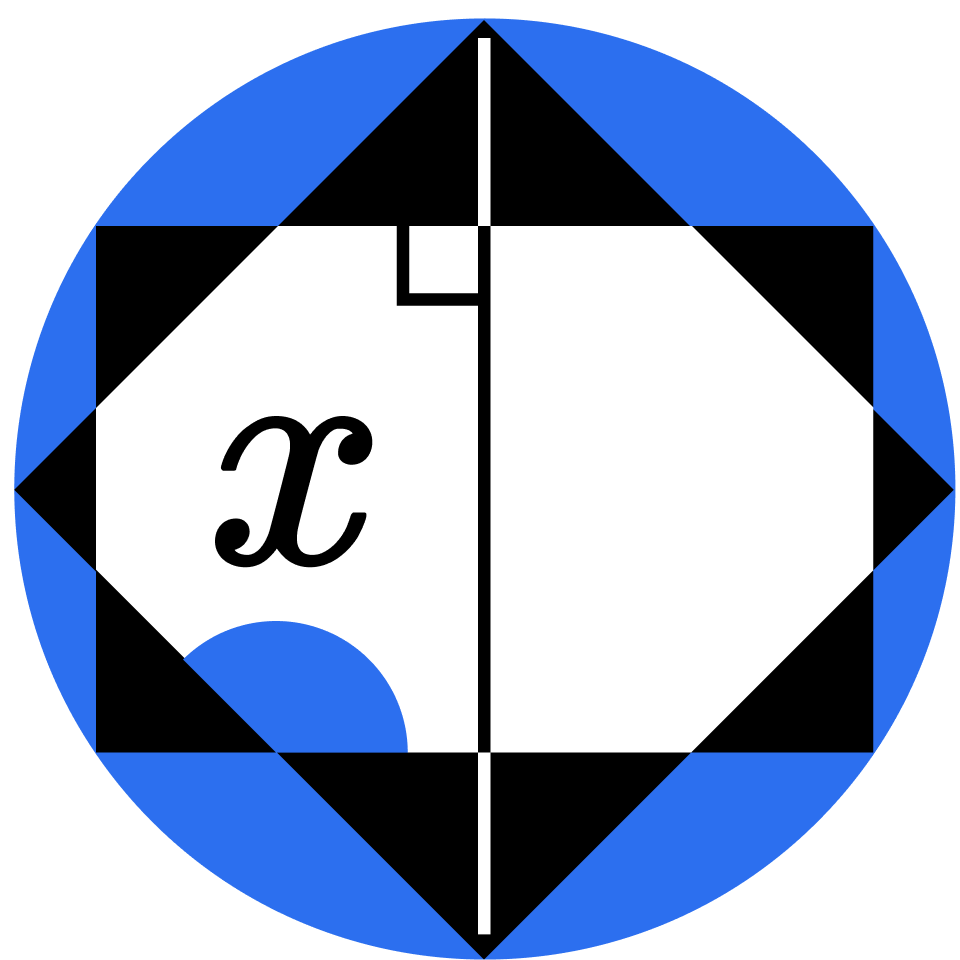## Geometry II

Continue on the road to geometry mastery with this proof-centric course.

Central Angles and Arcs

Thales's Theorem

Inscribed Angles

Puzzles with Inscribed Angles

Power of a Point I

Intersecting Secants

Power of a Point II

Tangents

Problem-Solving Challenges

Right Triangles

Thales + Pythagoras

Cevians

Pegboard Triangles

Three Different Centers

The Circumcenter

Euler's Line

Morley's Triangle

### Course description

This course covers a wide range of theorems in classical Euclidean geometry. You'll start by deriving the Central Angle Theorem and Thales' Theorem, then move on to the Power of a Point Theorem, and conclude with an exploration of different types of triangle centers and their presence on the Euler Line. Our goal is to help you understand and explore the derivations of these theorems, and to give you many opportunities to practice and strengthen your skills applying them! Algebra is used throughout this course, but nothing beyond the level of Algebra I. This course is a great place to start (or continue) if you're already familiar with the content in Geometry I.

### Topics covered

• Centroid
• Circumcenter
• Euler's Line
• Incenter
• Inscribed Angles
• Intersecting Chord Theorem
• Intersecting Secants Theorem
• Intersecting Tangent Theorem
• Orthocenter
• Power of a Point Theorem

• Geometry I